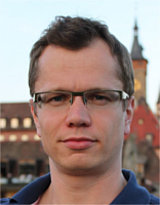# BARC talk by Joachim Spoerhase

Monday, 8 November 2021, Joachim Spoerhase from Würzburg University in Germany, will give a talk on "Constant-Factor Approximation for Ordered k-Median".

Title:
Constant-Factor Approximation for Ordered k-Median

Abstract:
We study the Ordered k-Median problem, in which the solution is evaluated by first sorting the client connection costs and then multiplying them with a predefined non-increasing weight vector (higher connection costs are taken with larger weights). Since the 1990s, this problem has been studied extensively in the discrete optimization and operations research communities and has emerged as a framework unifying many fundamental clustering and location problems such as k-Median and k-Center. Obtaining non-trivial approximation algorithms was an open problem even for simple topologies such as trees. Recently, Aouad and Segev (2017) were able to obtain an O(log n) approximation algorithm for Ordered k-Median using a sophisticated local-search approach. The existence of a constant-factor approximation algorithm, however, remained open even for the rectangular weight vector.

In this paper, we provide an LP-rounding constant-factor approximation algorithm for the Ordered k-Median problem.

We achieve this result by revealing an interesting connection to the classic k-Median problem. In particular, we propose a novel LP relaxation that uses the constraints of the natural LP relaxation for k-Median but minimizes over a non-metric, distorted cost vector. This cost function (approximately) emulates the weighting of distances in an optimum solution and can be guessed by means of a clever enumeration scheme of Aouad and Segev. Although the resulting LP has an unbounded integrality gap, we can show that the LP rounding process by Charikar and Li (2012) for k-Median, operating on the original, metric space, gives a constant-factor approximation when relating not only to the LP value but also to a combinatorial bound derived from the guessing phase. To analyze the rounding process under the non-linear, ranking-based objective of Ordered k-Median, we employ several new ideas and technical ingredients that we believe could be of interest in some of the numerous other settings related to ordered, weighted cost functions.

Authors: Jarosław Byrka, Krzysztof Sornat, Joachim Spoerhase

Bio:
You can find a short CV by clicking here.